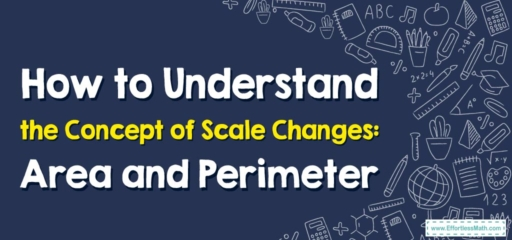# How to Understand the Concept of Scale Changes: Area and Perimeter

In the realm of geometry, understanding the concept of scale is crucial for accurate calculations and measurements. When it comes to determining the area and perimeter of shapes, scale changes play a significant role in altering their dimensions. This article delves into the fascinating world of scale changes in area and perimeter calculations. By mastering the art of scale changes, you will gain a deeper understanding of geometric properties and enhance your ability to accurately analyze and manipulate shapes.## A Step-by-step Guide to Understanding the Concept of Scale Changes: Area and Perimeter

Sure, let’s go through this concept step by step.

### Understand the Basic Concepts: Area and Perimeter

Perimeter: The perimeter is the length of the boundary of a two-dimensional shape. For example, in a rectangle, the perimeter can be calculated as twice the sum of length and breadth.

Area: The area is the amount of space inside a two-dimensional shape. For a rectangle, the area can be calculated as the product of its length and width.

### Understand the Concept of Scale

Scale is a ratio that compares the size of a model or map to the real world. For example, if a map’s scale is $$1:100,000$$, it means $$1$$ cm on the map represents $$100,000$$ cm (or $$1$$ km) in the real world. This concept can also be applied to geometric shapes.

### Effect of Scale on Perimeter

When we apply a scale factor to a two-dimensional shape, the perimeter will change by the same scale factor. For example, if we have a rectangle with a perimeter of $$12$$ units and we double the scale (scale factor of $$2$$), the new perimeter will be $$24$$ units.

In other words, if the length and width (or any other dimensions involved in the perimeter calculation) of a shape are multiplied by a scale factor of ‘$$k$$’, the perimeter of the new shape will be ‘$$k$$’ times the original perimeter.

### Effect of Scale on Area

The effect of scale on the area is a little different than its effect on the perimeter. When we scale a two-dimensional shape, the area will change by the scale factor squared. This means that if we double the scale (scale factor of $$2$$), the new area will be $$4$$ times the original area.

This occurs because the area is calculated by multiplying two dimensions together. So, if both dimensions are multiplied by a scale factor of ‘$$k$$’, the new area will be ‘$$k^2$$’ times the original area.

#### Examples

Suppose you have a rectangle with a length of $$3$$ units and a width of $$2$$ units. The perimeter is $$2(3+2) = 10$$ units and the area is $$3 \times 2 = 6$$ square units.
Now, if we increase the scale by a factor of 3, the new length is $$3 \times 3 = 9$$ units and the new width is $$2 \times 3 = 6$$ units. The new perimeter is $$2(9+6) = 30$$ units and the new area is $$9 \times 6 = 54$$ square units.
As you can see, the new perimeter is $$3$$ times the original perimeter, and the new area is $$9$$ (or $$3^2$$) times the original area.
Remember that this property holds true for all regular polygons, not just rectangles.

So that’s a step-by-step understanding of the topic ‘Area and Perimeter: Scale Changes’. Do let me know if you have any questions or if there’s anything you’d like to dive deeper into!

### What people say about "How to Understand the Concept of Scale Changes: Area and Perimeter - Effortless Math: We Help Students Learn to LOVE Mathematics"?

No one replied yet.

X
30% OFF

Limited time only!

Save Over 30%

SAVE $5 It was$16.99 now it is \$11.99